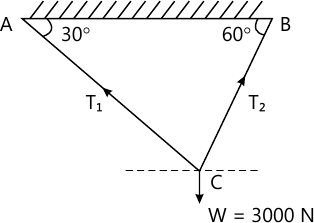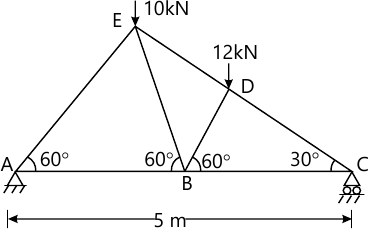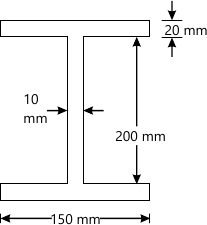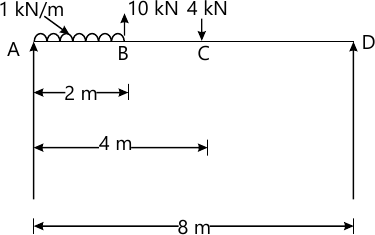MORE IN Basic Civil Engg. & Engg. Mechanics
RGPV First Year Engineering (Set B) (Semester 1)
Basic Civil Engg. & Engg. Mechanics
December 2012
Total marks: --
Total time: --
INSTRUCTIONS
(1) Assume appropriate data and state your reasons
(2) Marks are given to the right of every question
(3) Draw neat diagrams wherever necessary

Answer any one question from Q1 & Q2
1 (a) What is concrete? Describe the process of preparation of concrete.
7 M
1 (b) What are the various field tests applied on bricks to judge the quality of bricks?
7 M

2 (a) Draw the neat sketches of the following :
(i) King post roof truss.
(ii) Open Newel stairs.
7 M
2 (b) Explain the various elements of building construction.
7 M

Answer any one question from Q3 & Q4
3 (a) What are the various methods of plane table surveying? Explain any two in detail.
7 M
3 (b) Explain the EDM method in detail.
7 M

4 (a) Describe any two methods of measuring horizontal angle with theodolite.
7 M
4 (b) The following consecutive readings were taken with the help of dumpy level 1.905, 2.655, 3.905, 4.025, 1.965, 1.705, 1.595, 1.256, 2.545, 2.005, 3.145. The instrument was shifted after the fourth and seventh readings. The first reading was taken on the staff held on B.M. of R.L. 100.000 m. Rule out a page of level field book. Enter the above reading there on. Calculate the R.Ls of the point and apply the arithmetic check.
7 M

Answer any one question from Q5 & Q6
5 (a) Define 'contour'. What are the various characteristics of contour.
7 M
5 (b) The following perpendicular offset were taken at 20 m intervals from a base line to an irregular boundary line.
5.9, 12.4, 16.5, 15.3, 18.4, 20.9, 24.9, 21.8 and 19.2 meter. Calculate the area enclosed between the base line, the irregular boundary line and the first and last offset by-
(i) Trapezoidal Rule
(ii) Simpson's Rule
7 M

6 (a) Explain the term 'Remote sensing' in detail. What are the various applications of remote sensing.
7 M
6 (b) Explain the following in detail
(i) Survey stations.
(ii) Methods for measuring the area and volume.
7 M

Answer any one question from Q7 & Q8
7 (a) Define various types of forced with the help of sketches.
7 M
7 (b) A weight of 3000 N is supported by two chains AC and BC as shown in Fig. Below. Determine the tension in each chain.7 M

8 (a) State and explain the Traingle law and polygon law of forces.
7 M
8 (b) Determine the forces in all the members of the truss loaded and supported as shown in Fig. Below.7 M

Answer any one question from Q9 & Q10
9 (a) State parallel and perpendicular axis theorems. Explain any one in detail.
7 M
9 (b) Find the moment of intertia of a rolled steel joist girder of symmetrical I section shown in Fig below.7 M

10 (a) Define Various types of beams and loadings with the help of neat sketches.
7 M
10 (b) Draw the shear force and bending moment diagram for the loaded and supported as shown below.7 M

More question papers from Basic Civil Engg. & Engg. Mechanics